Research on combination forecast of port cargo throughput based on time series and causality analysis

Research on combination forecast of port cargo throughput based on time series and causality analysis

Chi Zhang, Lei Huang, Zhichao Zhao

School of Economics and Management, Beijing Jiaotong university (China)

Accepted January 2013

Zhang, C., Huang, L., & Zhao, Z. (2013). Research on combination forecast of port cargo throughput based on time series and causality analysis. Journal of Industrial Engineering and Management, 6(1), 124-134. http://dx.doi.org/10.3926/jiem.687

---------------------

Abstract:

Purpose: The purpose of this paper is to develop a combined model composed of grey-forecast model and Logistic-growth-curve model to improve the accuracy of forecast model of cargo throughput for the port. The authors also use the existing data of a current port to verify the validity of the combined model.

Design/methodology/approach: A literature review is undertaken to find the appropriate forecast model of cargo throughput for the port. Through researching the related forecast model, the authors put together the individual models which are significant to study further. Finally, the authors combine two individual models (grey-forecast model and Logistic-growth-curve model) into one combined model to forecast the port cargo throughput, and use the model to a physical port in China to testify the validity of the model.

Findings: Test by the perceptional data of cargo throughput in the physical port, the results show that the combined model can obtain relatively higher forecast accuracy when it is not easy to find more information. Furthermore, the forecast made by the combined model are more accurate than any of the individual ones.

Research limitations/implications: The study provided a new combined forecast model of cargo throughput with a relatively less information to improve the accuracy rate of the forecast. The limitation of the model is that it requires the cargo throughput of the port have an S-shaped change trend.

Practical implications: This model is not limited by external conditions such as geographical, cultural. This model predicted the port cargo throughput of one real port in China in 2015, which provided some instructive guidance for the port development.

Originality/value: This is the one of the study to improve the accuracy rate of the cargo throughput forecast with little information.

Keywords: cargo throughput, combined forecast model, Logistic growth curve model, Gray forecast model

---------------------

1. Introduction

By the impact of the continuing recession of the global economy, the trend of global port cargo throughput growth presented a significant slowdown in 2011. Compared with 14% growth rate in 2010, the global port cargo throughput was only about 7.3% growth rate in 2011, which reveal a large decline in global economy. Compared to most ports struggling to survive, the ports in China continued to maintain strong. Chinese port occupied seven seats among the first ten cargo throughput ports. Shanghai Port and Ningbo-Zhoushan Port are sustained the first and second largest cargo throughput port in the whole world.

Cargo throughput is very important for a port, it is not only the most basic production index for measuring the port development, but also a significant reference to organize its production, make its development plans and construction. In the meantime, the amount of the cargo throughput may reflect the economic situation and the development level of the port city. Cargo throughput forecast is a significant content of the port development strategy. The correctness and rationality of the forecast means much to various aspects in the development of ports including the scientific port layout, the scale of investment in infrastructure, business strategy, development strategy and the collection and distribution of integrated transport plan. This paper studies the forecast model of cargo throughput of the port, and then takes one port in China as an example to verify the model accuracy, in order to provide a strong reference to the port cargo throughput forecasts.

2. Analysis of current cargo throughput forecast model

Methods to predict the cargo throughput of the port can be summarized into two categories: qualitative and quantitative forecast methods. Qualitative forecast methods primarily use of historical data, and rely on personal experience, knowledge and analysis ability to predict the future development. It includes Delphi Method and the method of Survey on the Supply of Goods. Qualitative forecast methods have some defects such as the predict process lack of objectivity and the forecast are broad-brush. While quantitative forecast methods which built up by using mathematical statistical methods to predict the status of future is based on historical statistics. Owing to the more objective and meticulous feature quantitative forecast methods have, this paper would focus on a detailed classification and presentation of quantitative forecast methods.

Through the use of statistical methods and mathematical models, quantitative forecast methods based on accurate, timely, systematic, comprehensive survey of statistical data and economic information can predict the future of the port in size, level, speed and other variables (Wang & Yang, 2007; Xu, 2010). It is closely related to statistics, so quantitative forecast methods also known as the statistical prediction. Depending on the different mathematical methods used, quantitative forecast methods can be divided into three categories: time series, causality analysis, and combined forecast method.

2.1. Time series method

Time series method is to find out the variation law of the total port cargo throughput of historical data to establish a mathematical model to forecast. Such methods include: moving average, exponential smoothing, gray system, the seasonal changes, autoregressive method and time-independent variable regression analysis (Xu, 2011) method. For example, Jiang and Lei (2009) proved that nonlinear gray model is better than gray model to forecast the cargo throughput of Lianyungang Port; Xu (2011) used autoregressive forecast model to forecast the Shanghai Port cargo throughput. Guo, Song and Ye (2005) shows that the grey Verhulst model on time series error corrected is applicable. When the cargo throughput increases according to the curve with S type, not only higher forecasting accuracy can be obtained, but also the superiority and the features of grey system model can be reserved.

2.2. Causality analysis method

Causality analysis methods first identify the relationship between the cargo throughput and some hinterland economic indicators, and then establish the model based on these indicators. Finally this model will forecast the future of the port cargo throughput. Such methods include regression analysis, the coefficient of elasticity, the system dynamics method and the method of neural network (Lin & Chen, 2008). For instance, Huang, Cai and Yi (2010) took Levenberg-Marquardt algorithm into BP neural network, and then applied it to the port cargo throughput forecast. He found out forecast accuracy improved greatly by the Levenberg-Marquardt optimization model considering the factors of the national economy. Chen and Chen (2009) achieved promptly the accurate and satisfactory results in the Nanjing cargo throughput forecast with the BP Neural Network. Xu, Yan and Zhang (2006) made sure that the proposed model based on System Dynamics was verified to be effective and feasible by simulation using relevant statistical data of port volume of Ningbo City.

2.3. Combined forecast method

Combined forecast method doesn’t directly use of the historical and current data modeling, but establishes an appropriate combination of various kinds of individual forecast models to obtain the optimal (Zhang, 2007) value to forecast the port cargo throughput. For example, Chen and Gu (2010) combined gray model with the linear regression model which proved better than other alternatives. Wu and Feng (2011) first forecast the port cargo throughput with gray model, and then optimized the result by the Markov model in order to improve the accuracy of forecast.

3. The selection of combination forecast model

After the introduction above, we can easily find the combination forecast model can reduce the error of a single prediction model (Gao, 2008) to a larger extent. Some errors come from the reality of environmental factors, while others may exist problems in the process of modeling, so that would affect the accuracy of the model in predicting. This paper will make an organic combination of gray forecast model and Logistic population projection model, to achieve more accurate port forecast model.

3.1. Grey forecast model

The gray system theory (Deng, 2002; Sun & Zheng, 2007) is the study of how to solve the gray system analysis, modeling, forecasting, decision-making and control. It has been put forward and developed by Professor Deng Julong, Huazhong University of Science and Technology from 1982. In nearly three decades, this model has not only aroused the concern of many scholars, but also been developed by leaps and bounds. At present, it has become one of important method to forecast, decision-make, evaluate, plan and control, systems analyze and model in areas of society, economy, science and technology. Particularly, the gray system has a unique effect in analysis and modeling for short time series, statistics, and incomplete information systems, so it has been widely used.

Currently some of the commonly used forecast methods (such as regression analysis, etc.) require a larger number of samples. If the sample size is smaller, it often results in large deviation, which will make the forecast target fail. In the meantime, gray forecast model need less information and it is so convenient to operate that it becomes an effective tool to deal with small-sample forecast. It has been widely used in various fields for high-precise modeling. The forecast is according to the law of development of objective things, past and present, by means of scientific methods to describe and analyze future trends and conditions. Finally the forecast makes the formation of the scientific assumptions and judgments. Gray Forecast Model, abbreviated as GM (1, 1) (Liu, Jiang & Zhang, 2007), is as follows:

Firstly, there is an original sequence denoted by x(0), it is made up of x(0)(1), x(0)(2), …, x(0)(n). Then the original sequence is accumulated to obtain the generated sequence.“a”, “u” in the formula (2) are the undetermined coefficients sequences. We can arrange sequences as follows:According to the formula (4), (5), we can calculate the value of “a” and “u”, and then take them into the differential equation (2), so that the solution of the gray forecast model is: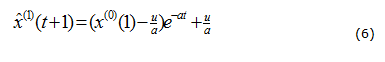The gray theory doesn’t establish original data model, but generate data model, so the gray theory forecast data does not come directly from the generated, but from the restored ones. We can restore the data in the formula (7).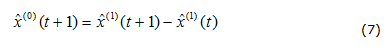In the formula (7), the equation of the gray forecast error is: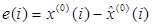, while the mean error is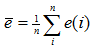.

3.2. Logistic growth curve model

Logistic curve model was first proposed by mathematician P.F.Veihulot in the study of the law of population growth. The model is characterized by a slow growth at first, then grows rapidly in a range, and slows down again after a certain limit reach, the curve presents as slightly elongated "S" shape. This is similar to the law of development of Chinese ports. Since reform and development in China, the economic growth continues, while the import and export volume and the domestic turnover has a huge upgrade in the port industry. However, after the 2008, influenced by the financial crisis, the development of the port industry in China also encountered a bottleneck. Cargo throughput of the port, as an important indicator to directly reflect the changes in the economic development of the port hinterland, just makes a good illustration of this trend. It is similar as what Logistic curve (Zhu, 2003) model wants to express, so we select this model as a reference.

Logistic curve equation is: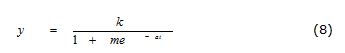In the equation (8), “t” stands for the point of the time sequence, generally natural numbers; “a”, “m” is the undetermined coefficient; “k” is a given saturation value by the forecasters according to the actual situation and the growing trend of “y”.

3.3. Combined forecast model

The greatest concern in the combined forecast (Ma, Wang & Li, 2009) is how to calculate the weighted mean coefficient to make the combined forecast model to improve the prediction accuracy. Suppose “m”, “n” is the coefficient, “m” stands for how many ways to forecast the cargo throughput, while “n” stands for how many time periods we use. yij(i=1,2,,n, j=1,2,,m) means the predictive value in the “j”th way and the “i”th time period. “rj” stands for the weight of the different forecast methods, it must be met .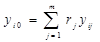Suppose yi0 as the combined predictive value in the “i”th time period, so that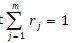.

Then establish the nonlinear (Li, Chen & Cui, 2008) programming in order to get minimum mean square error (MSE) as the objective function value:MSEj stands for the unbiased variance of the “j”th way.

4. A Port cargo throughput forecast

A Port in Southern China is an omnibus advocate hub port, driven by the rapid economic development of hinterland; the port cargo throughput continues to grow continuously. In 1999, the port cargo throughput exceeded 100 million tons, which made it become the second port into “the one hundred million tons club” in China. Since then, the port developed step by step. In 2011, the port cargo throughput reached 451 million tons, among the global port 4. The Port has 50 million-ton berths; 14 million-ton loading and unloading buoy; 23 million-ton loading and unloading of anchorage. Considered from any factors like the historical, economic and cultural, the Port is a representative in the ports of China and the world. With the Port cargo throughput of nearly 11 years as the sample, this paper studies the port cargo throughput prediction on combination forecasting model.

 year 2002 3 4 5 6 7 8 9 10 11 CT (Mt) 1532 1719 2152 2504 3028 3432 3470 3639 4110 4510

Table 1. The port cargo throughput of nearly 11 years (CT represents Cargo Throughput)

4.1. Establish GM (1, 1) model

According to the Port historical data, we can calculate through formula (1) for gray forecast model parameters: a = -0.1, u = 1567.7, while we can also get the small error probability is 1, variance is 0.1906. These two values ​​indicate that the model accuracy for A, so that we can use this result as forecast model:4.2. Establish Logistic growth curve model

Setting the initial value[x,y]= [1,18] in formula (2), we can get the undetermined coefficient “m” equals 4.225, “a” equals 0.2574. Finally, the Logistic growth curve equation is:(13)

4.3. Establish combined forecast model

Setting the weight of GM (1, 1) model, Logistic population model as y1, y2, we can calculate them from formula (3):r1=0.4975r2= 0.5025. Finally, combined forecast model is: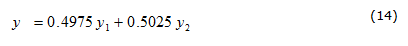4.4. Three forecast model comparison

There will always be some error due to many factors affect the port cargo throughput, when we use the various forecast methods to forecast. Therefore, evaluation of the various forecast methods, in order to improve forecast accuracy, has become an indispensable part of the forecast. This paper compares the error of three forecast methods to verify the superiority of the combined model.

 year original Logistic GM(1,1) combination (Mt) Fitted (Mt) error/% Fitted (Mt) error/% Fitted (Mt) error/% 2002 1532 1550.942 1.2 1797 17.2 1673.356 9.2 2003 1719 1850.679 7.6 1997.7 16.2 1923.822 11.9 2004 2152 2175.748 1.1 2220.9 3.2 2198.212 2.1 2005 2504 2517.617 0.5 2469 -1.3 2493.43 -0.4 2006 3028 2865.72 -5.3 2744.8 -9.3 2805.562 -7.3 2007 3432 3208.701 -6.5 3051.4 -11.1 3130.444 -8.8 2008 3470 3535.858 1.9 3392.2 -2.2 3464.388 -0.2 2009 3639 3838.411 5.4 3771.1 3.6 3804.924 4.5 2010 4110 4110.309 0 4192.4 2 4151.149 1 2011 4510 4348.437 -3.5 4660.7 3.3 4503.788 -0.1

Table 2. Comparison of three models

We can see that the combination forecast model is better than the others, and it is closer to the true value. This indicates that the combination forecast model during the port cargo throughput forecast is valid.

It can be seen from the above forecast: the combined forecast model is better than the individual forecast model. This indicates that the combined forecast method can improve the forecast accuracy and have a very good effect. The combined forecast method forecast that the port cargo throughput in 2015 will be 644 million tons. This will provide a basis for the Port to make the port development strategy.

5. Conclusion

As China grows in a stage of rapid development of urbanization, industrialization and economic globalization, the cargo throughput and container cargo throughput has maintained rapid growth over the years. The port facility capacity in China is so fast that the port construction may be developed in advance. However, if the capacity is serious surplus, not only its economic and social benefits cannot work, but will cause the waste of idle facilities and resources. Predicting the cargo throughput of the port is of great importance for state and local to formulate the port development strategy.

References

Chen, T.T., & Chen, Y.Y. (2009). Port Cargo throughput Forecast Based on BP Neural Network. Computer and Modernization, 10, 183.

Chen, X.Y., & Gu, H. (2010). Gray linear regression model in Port Cargo throughput Prediction. Port & Waterway Engineering, 5, 90-92.

Deng, J.L. (2002). Gray system theory tutorial. Huazhong University of Science and Technology, WuHan, China.

Gao, Y.(2008). The Selection of Prediction Model for Coal Port Cargo throughput of Qinhuangdao Port. Logistics Management, 2, 76-81.

Guo, Z.J., Song, X.Q., & Ye, J. (2005). A Verhulst model on time series error corrected for port cargo throughput forecasting. Journal of the Eastern Asia Society for Transportation Studies, 6, 881–891.

Huang, J., Cai Q.D., & Yi, W.X. (2010). LM-BP neural network-based coastal port cargo throughput Simulation and Prediction. Port & Waterway Engineering, 7, 63-65,103.

Jiang, F., & Lei, K. (2009). Grey Prediction of Port Cargo throughput Based on GM(1,1,a) Model. Logistics Technology, 9, 68-70.

Li, J.F., Chen, Y., & Cui, X.S. (2008). Port Cargo throughput Forecast Based on Nonlinear Combination Method. Proceedings of the 7th WSEAS Int. Conf. on Applied Computer & Applied Computational Science, 462-464.

Lin, Q., & Chen, Y.M. (2008). Neural network model applying in port cargo throughput forecasting and error analysis. Journal of Waterway and Harbor, 1, 89-91.

Liu, L.L., Jiang, H.Y., & Zhang, S.Z. (2007). Container cargo throughput forecast for Shenzhen Port based on GM(1,1) model. Port & Waterway Engineering, 2, 58-63.

Ma, X.C., Wang, X., & Li, H.F. (2009). Cargo throughput Forecast of Port Container Based on Combined Model. Communications Standardization, Z1, 104-107.

Sun, Y.M., & Zheng, G.P. (2007). Research on Forecast of Port Cargo throughput Capacity Based on Grey Theory. China Water Transport, 4, 144-147.

Wang, D., & Yang, Z. (2007). Analysis of Influential Factors of Port Cargo throughput. Port & Waterway Engineering, 1, 67-69.

Wu, Z., & Feng W. (2011). Grey Markov model-based port cargo throughput prediction research. China Water Transport, 11(8), 23-24.

Xu, C.X., Yan, Y.X., & Zhang, P. (2006). Prediction Model of Port Cargo throughput Based on System Dynamics. Port & Waterway Engineering, 5, 691.

Xu, J.H. (2010). Study on intrinsic factors of port cargo throughput based on principal component analysis. Port & Waterway Engineering, 1, 13-19.

Xu, Z.G. (2011). Based on the prediction of self-regression model of the Port of Shanghai port cargo throughput. Journal of Wuhan Technical College of Communications, 13(1), 71-74.

Zhang, H. (2007). Forecasting Method of Port Container Cargo throughput Based on Optimal Linear Composite Prediction Model. Journal of Wuhan University of Technology, 2, 82-85.

Zhu, Z.Y. (2003). A Comparative Study of the logistic curve and the Gompertz curve. Mathematics in Practice & Theory, 10, 66-71.